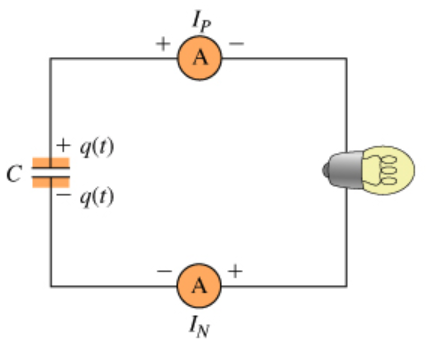# Problem: A large capacitor has a charge + on one plate and on the other. At time t = 0, the capacitor is connected in series to two ammeters and a light bulb. Immediately after the circuit is closed, the ammeter connected to the positive plate of the capacitor reads Ip and the ammeter connected to the negative plate of the capacitor reads IN. (Figure 1) Each ammeter reads positive if current flows through the circuit in a clockwise direction (from the + to the terminal of the meter).Part A Immediately after time t = 0, what happens to the charge on the capacitor plates? A) Individual charges flow through the circuit from the positive to the negative plate of the capacitor.B) Individual charges flow through the circuit from the negative to the positive plate of the capacitor. C) The positive and negative charges attract each other, so they stay in the capacitor. D) O Current flows clockwise through the circuit. E) Current flows counterclockwise through the circuit.Part B At any given instant after t = 0, what is the relationship between the current flowing through the two ammeters, Ip and In, and the current through the bulb, IB?A) Ip &gt; IB &gt; IN B) Ip = IB &gt;  IN C)  Ip &gt; IB  = IN D) Ip= IB = IN

###### FREE Expert Solution

Part A

The current in an external circuit is always directed away from the positive terminal towards the negative terminal of the battery.

90% (433 ratings)###### Problem Details

A large capacitor has a charge + on one plate and on the other. At time t = 0, the capacitor is connected in series to two ammeters and a light bulb. Immediately after the circuit is closed, the ammeter connected to the positive plate of the capacitor reads Ip and the ammeter connected to the negative plate of the capacitor reads IN. (Figure 1)

Each ammeter reads positive if current flows through the circuit in a clockwise direction (from the + to the terminal of the meter).Part A

Immediately after time t = 0, what happens to the charge on the capacitor plates?

A) Individual charges flow through the circuit from the positive to the negative plate of the capacitor.

B) Individual charges flow through the circuit from the negative to the positive plate of the capacitor.

C) The positive and negative charges attract each other, so they stay in the capacitor.

D) O Current flows clockwise through the circuit.

E) Current flows counterclockwise through the circuit.

Part B

At any given instant after t = 0, what is the relationship between the current flowing through the two ammeters, Iand In, and the current through the bulb, IB?

A) Ip > IB > IN

B) Ip = IB >  IN

C)  Ip > IB  = IN

D) Ip= I= IN